The Fridrich Method or the CFOP (Cross, F2L, OLL, PLL) method is a 3x3 and the most popular speedsolving method proposed by several cubers. This method mostly focuses on solving speed cube in a fast and efficient way. It is said that the average number of moves for the complete solution is around 56 moves.

The Fridrich method consists of only four steps –

1. Cross: Solving the first layer four edges
2. F2L: Solving the two layers completely
3. OLL: Orient the Last Layer correctly
4. PLL: Permute the Last Layer corner and edge pieces.

In this article we take a look at 2 Look OLL and 2 Look PLL.

## Notations to learn

 Face Notation Left Face L Right Face R Up Face U Down Face (bottom of the cube) D Front Face (the side facing you) F Back Face (the side facing away from you) B Middle Face (the centre ‘slice’) M Entire Cube Y

## Solving with 2 Look OLL

To orient the last layer, you need to focus on eight pieces. These eight pieces are the four corners and four edges.

2 Look OLL means that you will solve the OLL with two algorithms. The 2 Look OLL requires you to know ten algorithms that would already know if you can solve the 3x3 beginner's method.

1. Orientation of edges

This requires the use of one of these three algorithms.

Algorithm 1: When two opposite edges are present ,orient it as shown below.

 F R U R’ U’ F’Algorithm 2:
When two adjacent edges are present ,orient it as shown below

 F R U R' U' F'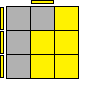Algorithm 3:
When no edges are oriented

 [F R U R' U' F'] x 21. Orientation of corners

This requires the use of 7 algorithms, as stated below.

Algorithm 1: Sune

 L U L' U [L U2 L']  (y2) R U R' U [R U2' R']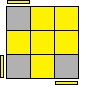Algorithm 2:
Anti-Sune

 R' U' R U' R' U2 R (y) R U2 R' U' R U' R'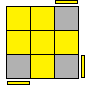Algorithm 3:

 R U2 R' U' R U R' U' R U' R' (y) R U R' U [R U' R' U] [R U2 R'] F [R U R' U'] [R U R' U'] [R U R' U'] F'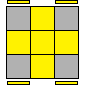Algorithm 4:
Superman

 R U2' [R2 U'] [R2 U'] R2 U2' R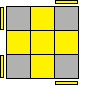Algorithm 5

 R2 D [R' U2 R] D' [R' U2 R']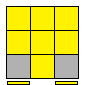Algorithm 6

 L' U' L U R U' [r' F]Algorithm 7

 [R' F R B'] [R' F' R B]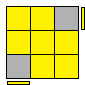## Solving with 2 Look PLL

There are 21 possible variations for permuting the last layer pieces. Compared to OLL, there are fewer algorithms to learn. However, you can solve the cube with just two algorithms.

1. Permuting the four corner pieces

Firstly, you need to find two adjacent correctly permuted corners. The best way to recognize this is by looking for two similar stickers on corner pieces. And then use the algorithm stated below:

 l’ U R’ D2 R U’ R’ D2 R2If you find two adjacent right corners, then perform this algorithm.

 x’ [R U’ R’ D] [R U R’ D’] [R U R’ D] [R U’ R’ D’]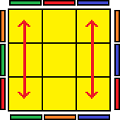1. Permuting the four edge pieces

After all the corner pieces are correctly permuted, there are only four possible variations for permuting the last layer edge pieces – Ua-perm, Ub-perm, Z-perm and H-perm.

Algorithm: Ua-Perm

 [R U' R] U R U R U' R' U' R2Algorithm:
Ub-Perm

 R2 U R U R' U' R' U' R' U R'Algorithm:
Z-perm

 M2 U M2 U M' U2 M2 U2 M' U2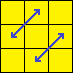Algorithm:
H-Perm

 M2 U M2 U2 M2 U M2By executing the suitable algorithms, you would have solved the 3x3 Rubik's cube completely.## Conclusion

Mastering this method also requires learning new algorithms. Since you are now familiar with the beginner's CFOP method, there are many advanced OLL and PLL algorithms that you can learn with advanced techniques. However, once mastered, you can speedsolving the Rubik's cube faster, and who knows, one day you might even break records!Taiburrahaman

Taiburrahaman Alipurduar westbangal India workTaiburrahaman

Taiburrahaman Alipurduar westbangal India workTaiburrahaman

Taiburrahaman Alipurduar westbangal India workno

No one reading this, that’s why Algorithm 1 and 2 are the same for years ;)Saroj Singh

3*3 and 4*4 cube is aussom cube so I want that and also a mirror cube